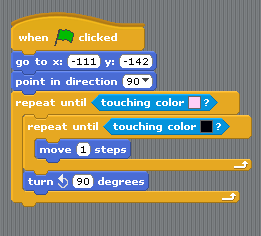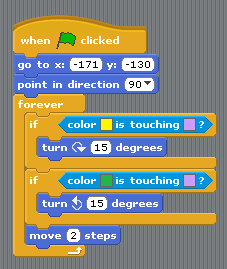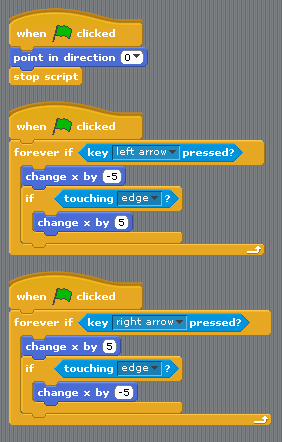# Scratch ProgrammingIteration

## Introduction

Iteration or repetition is the programming technique which allows you to have blocks of code repeatedly executed in your programs. We often refer to iteration structures in our programs as loops.

Scratch supports 3 basic types of iteration, all of which are explained on this page.

## Count-Controlled Loops

Count controlled loops repeat blocks of code a specific number of times.

### Example 1

This program outputs the word Hello! 3 times.Challenge: Copy the program and change it so that the user can choose how many times the message is repeated. Then make it so they can also enter the message that is to be repeated. You will need to make two variables to do this. The variables will need to be set before the loop starts and will be placed in the Repeat and Say blocks.

### Example 2

This program counts from 1 to 12 and outputs those numbers to the stage.Challenge 1: Copy and adapt the program so that it counts backwards.

Challenge 2: Copy and adapt the program so the user can choose where the counting starts. Set the value of the counter variable according to what they enter.

Challenge 3: Add another variable to the program so that the user enters 2 numbers before the loop starts. The program should count from the first number to the second number. You will need a third variable to work out how many times to repeat the loop. Subtracting the first number from the second number gives you the value you need.

Challenge 4: Write a new program that recites times tables. The user should be able to choose which tables to see. Use join blocks to output the information as you would when reciting a times table.

Challenge 5: Write a new program that outputs only even numbers. A number is even if it divides exactly by 2 - number mod 2 = 0. You will need to use an IF...Else block to do this.

Challenge 6: Write a new program that asks the user for a number and then reads out all of that number's factors. A number's factors are those numbers which divide exactly into that number. The following pseudocode describes how you might do this.

```Ask for the number Set number variable to what was entered Set factors variable to 1 and a space joined together Set counter variable to 2 Repeat number - 1 times    If number mod counter = 0 Then       Set factors to Join (factors, counter, space)    End If    Change counter by 1 End Repeat Output factors```

## Condition-Controlled Loops

Condition-controlled loops repeat blocks of code until a stopping condition is met.

### Example 3

This example is based on the second example but uses a Repeat Until block to achieve the same effect. Notice that you need to repeat the loop until the counter reaches 13, the stopping condition for this loop.Challenge 1: Change the example so that the user enters 2 numbers that will be the start and end of the counting. This is like the third challenge from the previous example. Set the counter to the value of the first number entered. Repeat the loop until the coutner reaches 1 more than the second number entered. Compare the program with the one you wrote for the previous challenge.

### Example 4

The program has the outline of a black rectangle drawn onto the stage along with a small pink zone. A car sprite travels rightwards until it meets a wall. It then turns 90° anti-clockwise and continues until it meets the next wall. When it reaches the pink area, the car stops moving. The stage looks like this,The script for this program involves nested loops is as follows,Challenge: Adapt this program by redesigning the stage to create a more interesting path from the start to the end. Remember that the car always turns left when it meets a wall.

## Continuous Loops

Scratch also has the facility for continuous loops. That is, the blocks are executed repeatedly. There are two such structures in Scratch, Forever and Forever If.

You have already met the Forever block in the page on selection.The track is the stage background. The car's wheels are all different colours. Here is the script that makes the car go around the track,### Example 5

This is the basic code to make a sprite move left and right when the arrow keys are pressed. Blocks are added to prevent the sprite from leaving the screen.The expression in the Forever If block checks to see if a particular key is pressed and responds accordingly.